2018-09-18  599 views 评论

# C++面向对象程序设计课程笔记（第八周）

## 第一节 string类

1.关于string

string类是模板类：typedef basic_string<char>string;实例出来的类。使用string类要包含头文件<string>。

string对象的初始化有以下几种类型：

string s1(“Hello);

string month = “March”;

string s2(8,’x’);

string error1 = 'c';//error

string error2('u');//error

string error3 = 22//error

string error4(22);//error

string s;

s = 'n';//OK

string对象的长度用成员函数length()读取，如下：

string s(“hello”);

cout<<s.length()<<endl;

string支持流读取运算符，如下：

string stringObject;

cin>> stringObject;

string支持getline函数：

string s;

getline(cin,s);

2.string的赋值和连接

（1）用=赋值：

string s1(“cat”),s2;

s2 = s1;

（2）用assign成员函数复制

string s1(“cat”),s3;

s3.assign(s1);

（3）用assign成员函数部分复制

string s1(“catpig”),s3;

s3.assign(s1,1,3);//从s1中下标为1的字符开始复制3个字符给s3

（4）单个字符复制

s2=s1=’a’;

（5）逐个访问string对象中的字符

string s1("Hello");

for(int i = 0;i<s1.length();i++)

cout<<s1.at(i)<<endl;

（6）用+运算符连接字符串

string s1(“good”),s2(“morning”);

s1+=s2;

cout<<s1;//输出goodmorning

（7）用成员函数append

s2.append(s1,3,s1.size());//s1.size()为s1的字符数

3.比较string

（1）用关系运算符比较string的大小：==/>/>=/</<=/!=

（2）用成员函数compare比较string的大小

string s1("hello"),s2("hello"),s3("hell");

int f1 = s1.compare(s2);//0

int f2 = s1.compare(s3);//1

int f3 = s3.compare(s1);//-1

int f4 = s1.compare(1,2,s3,0,3);//-1,比较s1的1-2与s3的0到3

int f5 = s1.compare(0,s1.size(),s3);//1，比较s1和s3的0-end

4.成员函数

（1）成员函数substr

string s1("hello world"),s2;

s2 = s1.substr(4,5);//下标4开始5个字符

cout << s2 <<endl;

（2）成员函数swap

string s1("hello"),s2("really");

s1.swap(s2);

（3）成员函数find()

string s1("hello world");

s1.find("lo");

（4）成员函数rfind()

string s1("hello world");

s1.rfind("lo");

（5）成员函数find_first_of()

string s1("hello world");

s1.find_first_of("abcd");

（6）成员函数find_last_of()

string s1("hello world");

s1.find_last_of("abcd");

（7）成员函数find_first_not_of()

string s1("hello world");

s1.find_first_not_of ("abcd");

（8）成员函数find_last_not_of()

string s1("hello world");

s1.find_last _not_of ("abcd");

（9）成员函数erase()。删除string中的字符。

（10）成员函数replace()。替换string中的字符。例如：

string s1("hello world");

s1.replace(2,3,"haha");

cout << s1;

//将s1下标2开始的三个字符替换为haha

（11）成员函数insert()。在string中插入字符。

string s1("hello world");

string s2("show insert");

s1.insert(5,s2); // 将s2插入s1下标5的位置

cout << s1 << endl;

s1.insert(2,s2,5,3);

//将s2中下标5开始的3个字符插入s1下标2的位置

cout << s1 << endl;

helloshow insert world

heinslloshow insert world

（12）成员函数c_str。转换成C语言式char*字符串

string s1(“hello world”);

printf(“%s\n,s1.c_str());//为了兼容C语言

// s1.c_str() 返回传统的const char * 类型字符串，且该字符串以‘\0’结尾。

（13）成员函数data()

string s1("hello world");

const char * p1=s1.data();

for(int i=0;i<s1.length();i++)

printf("%c",*(p1+i));

//s1.data() 返回一个char * 类型的字符串，对s1 的修改可能会使p1出错。

（14）成员函数copy()。字符串拷贝函数。

string s1("hello world");

int len = s1.length();

char * p2 = new char[len+1];

s1.copy(p2,5,0);

p2=0;

cout << p2 << endl;

// s1.copy(p2,5,0) 从s1的下标0的字符开始制作一个最长5个字符长度的字符串副本并将其赋值给p2。返回值表明实际复制字符串的长度。

5.字符串流处理

#include <string>

#include <iostream>

#include <sstream>

string input("Input test 123 4.7 A");

istringstream inputString(input);

string string1, string2;

int i;

double d;

char c;

inputString >> string1 >> string2 >> i >> d >> c;

cout << string1 << endl << string2 << endl;

cout << i <<endl << d << endl << c << endl;

long L;

if(inputString >> L)

cout << "long\n";

else cout << "empty\n";

Input test 123 4.7 A empty

ostringstream outputString;

int a = 10;

outputString << "This " << a << " ok" << endl;

cout << outputString.str();

## 第二节 标准模板库STL概述（一）

1.泛型程序设计

C++语言的核心优势之一就是便于软件的重用。C++中有两个方面体现重用：

（1）面向对象的思想：继承和多态，标准类库。

（2）泛型程序设计的思想：模板机制，以及标准模板库STL。

2.STL中的基本概念

sort(array,array+70);

3.容器概述

①顺序容器：vector，deque，list。

②关联容器：set，multiset，map，multimap。

③容器适配器：stack，queue，priority_queue。

（1）顺序容器

①vector   头文件<vector>

②deque 头文件<deque>

③list 头文件<list>

（2）关联容器

①set/multiset 头文件<set>

set即集合。set中不允许相同元素，multiset中允许相同的元素。

②map/multimap 头文件<map>

map与set的不同在于map中存放的元素有且仅有两个成员变量，一个名为first，另一个名为second，map根据first值对元素进行从小到大排序，并可快速根据first来检索元素。

map同multimap的不同在于是否允许相同first值的元素。

（3）容器适配器

①stack：头文件 <stack>

②queue 头文件<queue>

③priority_queue 头文件<queue>

4.顺序容器和关联容器中都有的成员函数

 成员函数 功能 begin 返回指向容器中第一个元素的迭代器。 end 返回指向容器中最后一个元素后面的位置的迭代器。 rbegin 返回指向容器中最后一个元素的迭代器。 rend 返回只想容器中第一个元素前面的位置迭代器。 erase 从容器中删除一个或几个元素。 clear 从容器中删除所有元素。

5.顺序容器的常用成员函数

 成员函数 功能 front 返回容器中第一个元素的引用。 back 返回容器中最后一个元素的引用。 push_back 在容器末位增加新元素。 pop_back 删除容器模块的元素。 erase 删除迭代器指向的元素(可能会使该迭代器失效），或删除一个区间，返回被删除元素后面的那个元素的迭代器。

## 第三节 标准模板库STL概述（二）

1.迭代器

（1）基本概念

• 用于指向顺序容器和关联容器中的元素
• 迭代器用法和指针类似
• 有const 和非 const两种
• 通过迭代器可以读取它指向的元素
• 通过非const迭代器还能修改其指向的元素

（2）迭代器的定义

*迭代器变量名

#include <vector>

#include <iostream>

using namespace std;

int main() {

vector<int> v; //一个存放int元素的数组，一开始里面没有元素

v.push_back(1);v.push_back(2);v.push_back(3);v.push_back(4);

vector<int>::const iterator i;//常量迭代器

for(int i = v.begin();i!=v.end;++i)

cout<<*i<<",";

cout<<endl;

//输出结果1,2,3,4,

vector<int>::reserve.iterator r;//反向迭代器

for(r = v.rbegin();r!=v.rend();r++)

cout<<*r<<",";

cout<<endl;

//输出结果：4,3,2,1,

vector<int>::iterator j;//非常量迭代器

for(j = v.begin();j!=v.end();j++)

*j = 100;

for(i = v.begin();i!=v.end();i++)

cout<<*i<<",";

//输出结果：100,100,100,100,

return 0;

}

（3）双向迭代器

++p,p++：使p指向容器中下一个元素；

--p,p--：使p指向容器的上一个元素；

*p：取p指向的元素；

p = p1：赋值；

p==p1,p!=p1：判断是否相等

（4）随机访问迭代器

p += i 将p向后移动i个元素

p -= i 将p向向前移动i个元素

p + i 值为: 指向 p 后面的第i个元素的迭代器

p - i 值为: 指向 p 前面的第i个元素的迭代器

p[i] 值为: p后面的第i个元素的引用

p < p1, p <= p1, p > p1, p>= p1

p – p1  : p1和p之间的元素个数

 容器 容器上的迭代器类别 vector 随机访问 deque 随机访问 list 双向 set/multiset 双向 map/multimap 双向 stack 不支持 queue 不支持 priority_queue 不支持

vector<int> v(100);

int i;

for(i = 0;i < v.size() ; i ++)

cout<<v[i];//根据下标随机访问

vector<int>::const_iterator ii;

for( ii = v.begin(); ii != v.end ();++ii)

cout << * ii;

for( ii = v.begin(); ii < v.end ();++ii )

cout << * ii;

list<int> v;

list<int>::const_iterator ii;

for(ii=v.begin();ii!=v.end();++ii)

cout<<*ii;

2.算法

template<class InIt, class T>

InIt find(InIt first, InIt last, const T& val);

first 和 last 这两个参数都是容器的迭代器，它们给出了容器中的查找区间起点和终点[first,last)。区间的起点是位于查找范围之中的，而终点不是。find在[first,last)查找等于val的元素。用 == 运算符判断相等。函数返回值是一个迭代器。如果找到，则该迭代器指向被找到的元素。如果找不到，则该迭代器等于last。

#include <vector>

#include <algorithm>

#include <iostream>

using namespace std;

int main()  {

int array = {10,20,30,40};

vector<int> v;

v.push_back(1);v.push_back(2);v.push_back(3);v.push_back(4);

vector<int>::iterator p;

p = find(v.begin(),v.end(),3);

if(p!=v.end())

cout<<*p<<endl;//输出3

p = find(v.begin(),v.end(),9)

if(p == v.end())

p = find(v.begin+1,v.end()-2,1);

//查找区间为[2,3)

if(p!=v.end())

cout<<*p<endl;//输出结果为3，因为找不到就返回v.end()，在这里相当于v.end()的是3这个位置

int *pp = find(array,array+4,20);//数组名是迭代器

cout<<*pp<<endl;//输出20

}

3.STL中的“大”、“小”和“相等”

（1）x比y小；

（2）表达式“x<y”为真；

（3）y比x大。

## 第四节 vector，deque和list

1.vector

vector示例程序1

#include <iostream>

#include <vector>

using namespace std;

template<class T>

void PrintVector( T s, T e){

for(; s != e; ++s)

cout << * s << " ";

cout << endl;

}

int main(){

int a = {1,2,3,4,5};

vector<int> v(a,a+5);//将数组a的内容放入v

cout<<"1)"<<v.end()-v.begin()<<endl;

//两个随机迭代器可以相减，输出1)5

cout<<"2)";

PrintVector(v.begin(),v.end());

//2)1 2 3 4 5

v.insert(v.begin()+2,13);

cout<<"3)";

PrintVector(v.begin(),v.end());

//3)1 2 13 3 4 5

v.erase(v.begin()+2);

cout<<"4)";

PrintVector(v.begin(),v.end());

//4)1 2 3 4 5

vector<int> v2(4,100);//v2有4个元素，都是100

v2.insert(v2.begin(),v.begin()+1,v.begin()+3);

cout<<"5)";

PrintVector(v2.begin(),v2.end());

//5)2 3 100 100 100 100

v.erase(v.begin() + 1, v.begin() + 3);

//删除 v 上的一个区间,即 2,3

cout << "6) ";

PrintVector(v.begin(),v.end());

//6) 1 4 5

return 0;

}

#include <iostream>

#include <vector>

using namespace std;

int main()

{

vector<vector<int> > v(3);

//v有3个元素，每个元素都是vector<int>容器

for(int i = 0;i<v.size();++i)

for(int j = 0;j<4;++j)

v[i].push_back(j);

for(int i = 0;i<v.size();++i){

for(int j = 0;j<v[i].size();++j)

cout<<v[i][j]<<" ";

cout<<endl;

}

return 0;

}

2.deque

deque还有 push_front（将元素插入到前面） 和pop_front(删除最前面的元素）操作，复杂度是O(1)。

3.双向链表

 函数名 作用 函数名 作用 push_front 在前面插入 unique 删除所有和前一个元素相同的元素（要做到元素不重复，则在unique之前还需要sort） pop_front 删除最前面的元素 merge 合并两个链表，并清空被合并的那个 sort 排序（但不支持STL的算法sort） reverse 颠倒链表 remove 删除和指定值相等的所有元素 splice 在指定位置前面插入另一链表中的一个或多个元素，并在另一链表中删除被插入的元素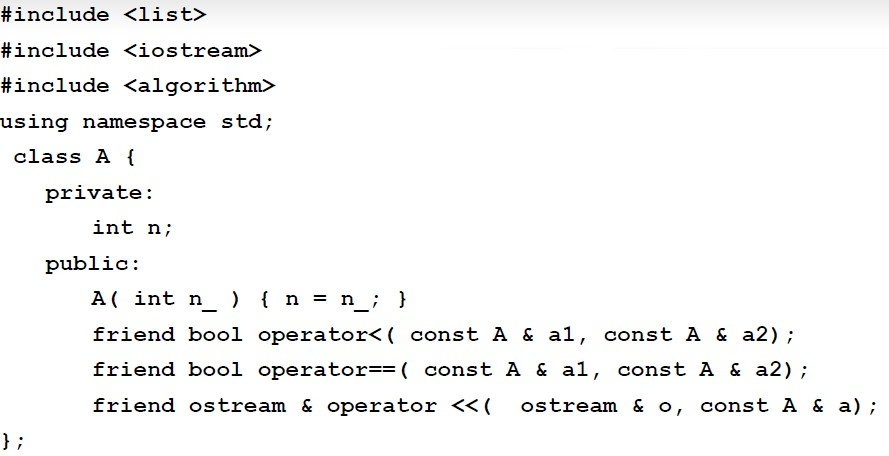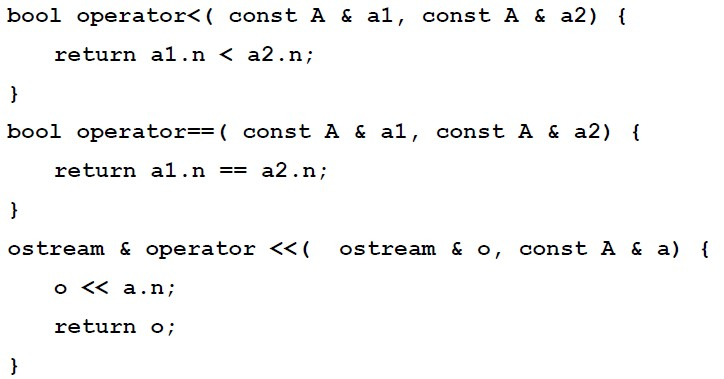template <class T>

void PrintList(const list<T> & lst) {

//不推荐的写法，还是用两个迭代器作为参数更好

int tmp = lst.size();

if( tmp > 0 ) {

typename list<T>::const_iterator i;

i = lst.begin();

for( i = lst.begin();i != lst.end(); i ++)

cout<<*i<<",";

}

}//typename用来说明 list<T>::const_iterator是个类型 //在vs中不写也可以

int main(){

list<A> lst1,lst2;

lst1.push_back(1);lst1.push_back(3); lst1.push_back(2);lst1.push_back(4);

lst1.push_back(2);

lst2.push_back(10);lst2.push_front(20);

lst2.push_back(30);lst2.push_back(30);

lst2.push_back(30);lst2.push_front(40); lst2.push_back(40);

cout<<"1)";PrintList(lst1);cout<<endl;

//1)1,3,2,4,2

cout<<"2)";PrintList(lst2);cout<<endl;

//2)40,20,10,30,30,30,40

lst2.sort();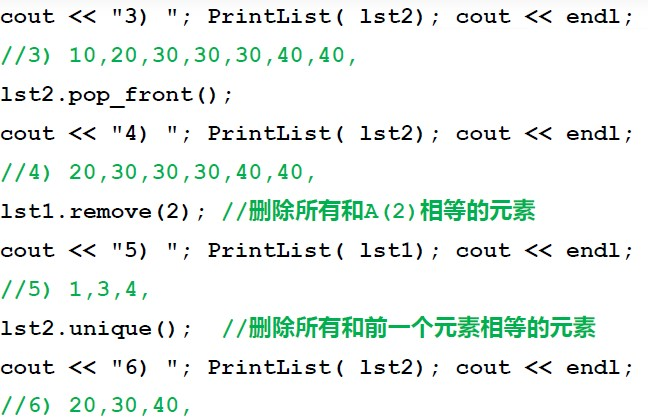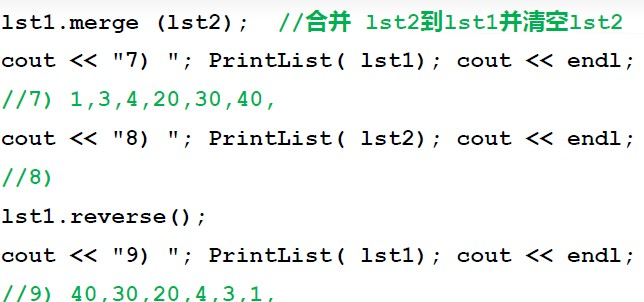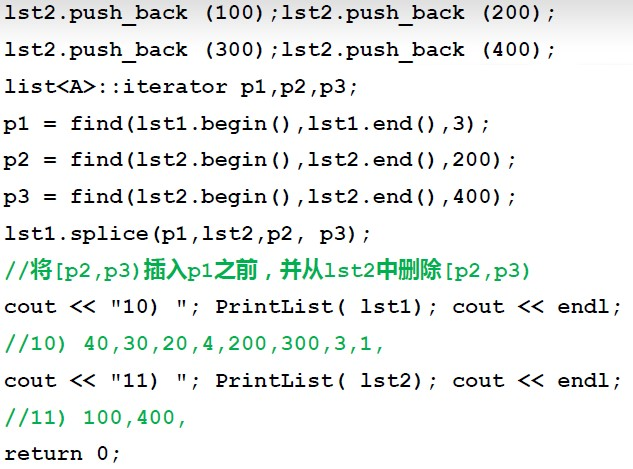## 第五节 函数对象

1.函数对象定义

class CMyAverage{

public:

double operator()(int a1, int a2, int a3){

return (double)(a1+a2+a3)/3;

}

};

CMyAverage agerage;

cout<<agerage(3,4,5);//输出为4

2.STL里有模板举例

（1）template<class InIt, class T, class Pred>

T accumulate(InIt first, InIt last, T val, Pred pr);

pr就是一个函数对象，对[first,last)中的每个迭代器I，执行val = pr(val,*I),返回最终的val。

pr也可以是个函数。

Dev C++中的Accumulate源代码1：

template<typename _InputIterator, typename _Tp>

_Tp accumulate(_InputIterator __first, _InputIterator __last, _Tp __init){

for(;__first!=__last;++__first)

__init = __init + *__first;

return __init;

}

//typename等价于class

Dev C++中的Accumulate源代码2：

template<typename _InputIterator, typename _Tp, typename _BinaryOperation>

_Tp accumulate(_InputIterator __first, _InputIterator __last, _Tp __init,_BinaryOperation __binary_op){

for(;__first!=__last;++__first)

__init = __binary_op(__init + *__first);

return __init;

}

（2）greater函数对象类模板

template<class T>

struct greater : public binary_function<T, T, bool> {

bool operator()(const T& x, const T& y) const {

return x>y;

}

};

list有两个sort函数，第一个是前面所说的不带参数的sort函数，它将list中的元素按“规定的比较方法”升序排列。

list还有另一个sort函数：

template <class T2>

void sort(T2 op);

#include <list>

#include <iostream>

#include <iterator>

using namespace std;

class MyLess {

public:

bool operator()(const int & c1, const int & c2)

{

return (c1%10)<(c2%10);

}

};

int main()

{

const int SIZE = 5;

int a[SIZE] = {5,21,14,2,3};

list<int> lst(a,a+SIZE);

lst.sort(MyLess()); //调用的第二种sort排序，由MyLess()来确定，准确的说是()确定

Print(lst.begin(),lst.end());

cout<<endl;

lst.sort(greater<int>());//greater<int>()是个对象

Print(lst.begin(),lst.end());

cout<<endl;

return 0;

}

21,14,5,3,2,（倒序排列）

3.STL中使用自定义的“大”“小”关系

①x小于y；

②op(x,y)返回值为true；

③y大于x。

#include <iostream>

#include <iterator>

using namespace std;

class MyLess {

public:

bool operator()(const int & c1, const int & c2)

{

if((c1%10)<(c2%10))

return true;

elsereturn false;

}

bool MyCompare(int a1, int a2)

{

if((a1%10)<(a2%10))

return false;

else

return true;

}

};

template <class T, class Pred>

T MyMax(T first, T last, Pred myless)

{

T tmpMax= first;//tmpMax是一个迭代器

for(;first!=last;++first)

if(myless(*tmpMax,*first))

tmpMax = first;

return tmpMax;

};

int main()

{

int a[] = {35,7,13,19,12};

cout<<*MyMax(a,a+5,MyLess())<<endl;//个位数最大

cout<<*MyMax(a,a+5,MyCompare)<<endl;//个位数最小

return 0;

}